Courses

# Class 12 Notes | EduRev

## JEE : Class 12 Notes | EduRev

The document Class 12 Notes | EduRev is a part of the JEE Course Physics Class 12.
All you need of JEE at this link: JEE

Effect of Temperature on Resistance

(a) Resistance of Pure Metals

(i) We know thatFor a given conductor, l, A and n are constant, hence R  is directly proportional to (1/τ)

If λ represents the mean free path (Average distance covered between two successive collisions) of the electron and vrms, the root-mean-square speed, then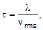Hence R is directly proportional to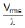Now,

(a) λ decreases with rise in temperature because the amplitude of vibrations of the +ve ions of the metal increases and they create more hindrance in the movement of electrons and,

(b) (i) vrms increases because vrms is directly proportional to  under root T. Therefore, Resistance of the metallic wire increases with rise in temperature. As ρ is directly proportional to  R and σ is directly proportional to (1/ρ), hence resistivity increases and conductivity decreases with rise in temperature of the metallic of the metallic wires.

(ii) If R0 and Rτ represent the resistances of metallic wire at 0°C and t°C respectively then Rt is given by the following formula :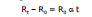where α is called as the Temperature coefficient of resistance of the material of the wire.

α depends on material and temperature but generally it is taken as a constant for a particular material for small change.

Rt - R0 = R0 α t

for very small change in temperature dR = R0 α dt

(c) Resistance of semiconductors

(i) There are certain substances whose conductivity lies in between that of insulators and conductors, higher than that of insulators but lower than that of conductors. These are called as semiconductors, e.g., silicon, germanium, carbon etc.

(ii) The resistivity of semiconductors decreases with increase in temperature i.e., a for semiconductors is -ve and high.

(iii) Though at ordinary temperature the value of n (no. of free electrons per unit volume) for these materials is very small as compared to metals, but increases very rapidly with rise in temperature (this happens due to breaking of covalent bonds). Though τ decreases but factor of n dominates. Therefore, the resistance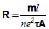goes on decreasing with increase in temperature.

5. Battery and EMF

A battery is a device which maintains a potential difference between its two terminals A and B. Figure shows a schematic diagram of a battery. Some internal mechanism exerts forces on the charges of the battery material. This force drives the positive charges of the battery material towards A and the negative charges of the battery material towards B. We show the force on a positive charge q as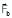. As positive charge accumulates on A and negative charge on B, a potential difference develops and grows between A and B. An electric field is developed in the battery material from A to B and exerts a force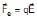on a charge q. The direction of this force is opposite to that ofIn steady state, the charge accumulation on A and B is such that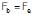. No further accumulation takes place.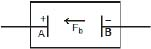If a charge q is taken from the terminal B to the terminal A , the work done by the battery force Fb is
W = Fb d where d is the distance between A and B. The work done by the battery force per unit charge is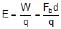This quantity is called the emf of the battery. The full form of emf is electromotive force. The name is misleading in the sense that emf is not a force, it is work done/charge. We shall continue to denote this quantity by the short name emf. If nothing is connected externally between A and B,

Fb = Fe = qE

or, Fbd = qEd = qV

where V = Ed is the potential difference between the terminals. Thus,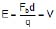Thus, the emf of a battery equals the potential difference between its terminals when the terminals are not connected externally.

Potential difference and emf are two different quantities whose magnitudes may be equal in certain conditions. The emf is the work done per unit charge by the battery force Fb which is non-electrostatic in nature. The potential difference originates from the electrostatic field created by the charges accumulated on the terminals of the battery.

A battery is often prepared by putting two rods or plates of different metals in a chemical solution. Such a battery, using chemical reactions to generate emf, is often called a cell.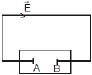Now suppose the terminals of a battery are connected by a conducting wire as shown in above figure. As the terminal A is at a higher potential than B, there is an electric field in the wire in the direction shown in the figure. The free electrons in the wire move in the opposite direction .and enter the battery at the terminal A. Some electrons are withdrawn from the terminal B which enter the wire through the right end. Thus, the potential difference between A and B tends to decrease. If this potential difference decreases, the electrostatic force Fe inside the battery also decreases. The force Fb due to the battery mechanism remains the same. Thus, there is a net force on the positive charges of the battery material from B to A. The positive charges rush towards A and neutralize the effect of the electrons coming at A from the wire. Similarly, the negative charges rush towards B. Thus, the potential difference between A and B is maintained.

For calculation of current, motion of a positive charge in one direction is equivalent to the motion of a negative charge in opposite direction. Using this fact, We can describe the above situation by a simpler model. The positive terminal of the battery supplies positive charges to the wire. These charges are pushed through the wire by the electric field and they reach the negative terminal of the battery. The battery mechanism drives these charges back to the positive terminal against the electric field existing in the battery and the process continues. This maintains a steady current in the circuit

Current can also be driven into a battery in the reverse direction. In such a case, positive charge enters the battery at the positive terminal, moves inside the battery to the· negative terminal and leaves the battery from the negative terminal. Such a process is called charging of the battery. The more common process in which. the positive charge comes out of the battery from the positive terminal is called discharging of the battery.

Ex.8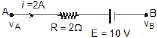Find vA - vB

Sol. vA - iR - E = vB

vA - vB = iR E = 4 +10 = 14 volt

Ex.9 Shown in the figure. Find out the current in the wire BD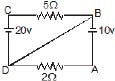Sol. Let at point D potential = 0 and write the potential of other points then current in wire AD =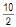= 5A from A to D current in wire CB =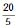= 4A from C to B. Therefore, current in wire BD = 1 A from D to B.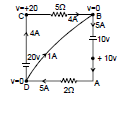Ex.10 Find the current in each wire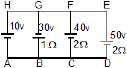Sol. Let potential at point A is 0 volt then potential of other points is shown in figure.

Current in BG =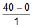= 40 A from G to B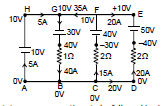Current in FC =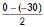= 15A from C to K

current in DE =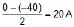from D to E

current in wire AH = 40 - 35 = 5 A from A to H

6. Kirchhoff's Laws for Circuit Analysis

Before moving on to the statement of Kirchhoff's law, we state some conventions to be followed in circuit analysis :

(1) Direction of conventional current is from high potential to low potential terminal.

(2) Current flows from high potential node A to low potential node B. if we traverse from point A to B, there is drop of potential; similarly from B to A, there is gain of potential.

If we traverse from point A to B, there is drop of potential; similarly from B to A, there is gain of potential. If a source of emf is traversed from negative to positive terminal, the change in potential is +E.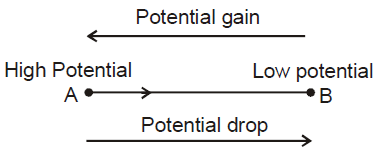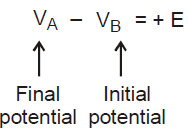While discharging, current is drawn from the battery, the current comes out from positive terminal and enters negative terminal, while charging of battery current is forced from positive terminal of the battery to negative terminal. Irrespective of direction of current through a battery the sign convention mentioned above holds.

The positive plate of a capacitor is at high potential and negative plate at low potential. If we traverse a capacitor from positive plate to negative plate, the change in potential is -Q/C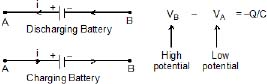If we traverse a resistor in the direction of current, the change in potential is -IR.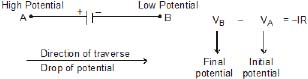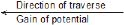If we traverse a resistor in the direction opposite to the direction of current, the change in potential is +IR.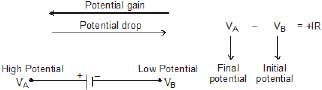Positive terminal of source of emf is at high potential and negative terminal at low potential. If we traverse a source of emf from the positive terminal to negative terminal, the change in potential is -E.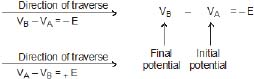If a capacitor is traversed from negative plate to positive plate, the change in potential is Q/C.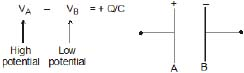(a) The Kirchhoff's Current Law

Current flow in circuits is produced when charge carriers travel though conductors. Current is defined as the rate at which this charge is carried through the circuit. A fundamental concept in physics is that charge will always be conserved. In the context of circuits this means that, since current is the rate of flow of charge, the current flowing into a point must be the same as current flowing out of that point.Kirchhoff's current law states that for the diagram above, the currents in the three wires must be related by:

𝐼1+𝐼2=𝐼3I1+I2=I3

It is important to note what is meant by the signs of the current in the diagram - a positive current means that the currents are flowing in the directions indicated on the diagram.

Offer running on EduRev: Apply code STAYHOME200 to get INR 200 off on our premium plan EduRev Infinity!

,

,

,

,

,

,

,

,

,

,

,

,

,

,

,

,

,

,

,

,

,

;